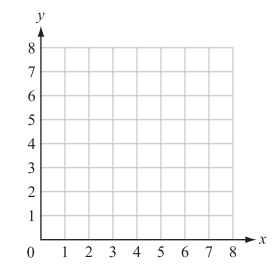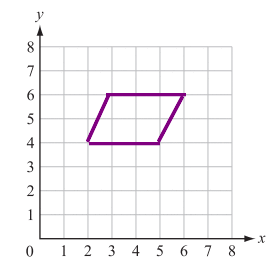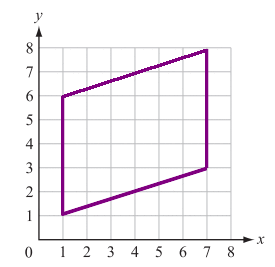# ISEE Lower Level Math : How to find a parallelogram on a coordinate plane

## Example Questions

### Example Question #1 : How To Find A Parallelogram On A Coordinate Plane

A coordinate plane is shown.Ralph plotted the following points on the coordinate grid:

Point W (2, 4); Point X (3, 6); Point Y (5, 4); Point Z (6, 6)

A polygon is formed with vertices W, X, Y, and Z. Which type of polygon is formed?

Parallelogram

Pentagon

Trapezoid

Kite

Square

Parallelogram

Explanation:

Begin by plotting the points and connecting the vertices.The quadrilateral that is created has two sets of parallel sides. Out of the possible answer choices, this can describe both squares and parallelograms. Because the figure does not contain right angles and the sides are not all the same length, it must be a parallelogram.

### Example Question #2 : How To Find A Parallelogram On A Coordinate Plane

A coordinate plane is shown.Ralph plotted the following points on the coordinate grid:

Point W (1, 1); Point X (7, 3); Point Y (1, 6); Point Z (7, 8)

A polygon is formed with vertices W, X, Y, and Z. Which type of polygon is formed?

Triangle

Rhombus

Parallelogram

Trapezoid

Hexagon

Parallelogram

Explanation:

Begin by plotting and connecting the vertices.The quadrilateral that is created has two sets of parallel sides. Out of the possible answer choices, this can describe both rhombuses and parallelograms. Because the sides are not all the same length, it must be a parallelogram.